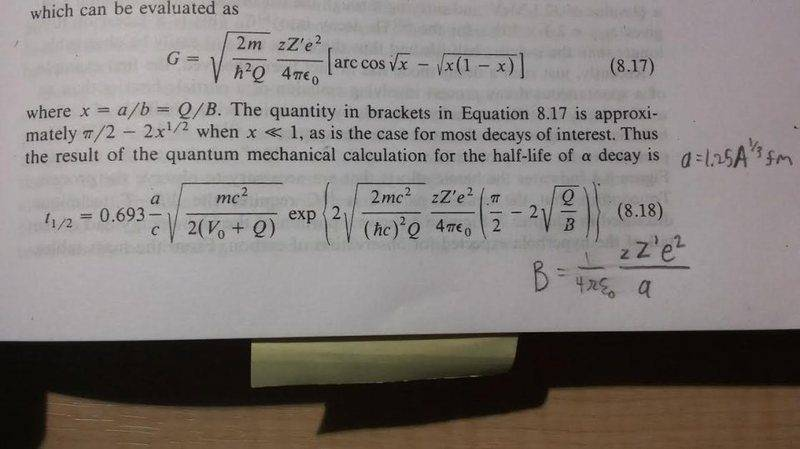# How do I use this equation? Estimating half-life of a decay

• llatosz

#### llatosz

Moved from technical forums, missing template
How should I go about using equation 8.18? Link can be found below. In the book, an example is used where
Th-220 --> C-12 + Po-208 with a Q value of 32.1 MeV is used, and it is said to yield
t1/2 = 2.3x106 but for the life of me I cannot reproduce this result. This is what I did:
Going from left to right in the equation,
-for a/c i used (1.25*2201/3 x10^-12 m ) / (3x10^8 m/s)
-for V0 I used 35 MeV which was also given
-for mc^2 I used amu * 931.5 MeV/(amu * c^2)
-for h-bar * c i used ( 6.582x10^-10 MeV * s ) * (3x10^8 m/s)
-for z i used 6
-for Z' i used 84
-for 1/(4πε0) i used 8.988x10^9 Nm^2/c^2I used all SI units except for my MeV which cancel. But I keep getting an overflow error in my Ti-84 Plus. What could be wrong?

-for a/c i used (1.25*2201/3 x10^-12 m ) / (3x10^8 m/s)
Here's one problem (there may be others): 1 fm = 10-15 m, not 10-12 m. If you used the same value of a when calculating B, you need to fix it there, also; this might have a big effect because it's inside an exponential.

Ah you're right! But unfortunately it still didn't work. I calculated all the terms separately and that exponent is just way too high no matter what. I might just call this one a loss lol. I really appreciate your reply though!

I used all SI units except for my MeV which cancel.

Yep, I used amu in order to be able to use the 931.5 MeV/(amu*c^2) too

Can you pin down which is the big number? For instance, the text above equation (8.18) indicates that x = Q/B should be << 1. Is it?

I just noticed that eq. (8.18) is for alpha-decay, not 12C decay; does that make a difference?

I just noticed that eq. (8.18) is for alpha-decay, not 12C decay; does that make a difference?
It shouldn't make a difference because this equation is flexible for any massive decay. For alpha decay, the difference between z and Z' would be 2, for 12C decay, z-Z' would be 6

Can you pin down which is the big number? For instance, the text above equation (8.18) indicates that x = Q/B should be << 1. Is it?
I remember the first multiplication term in the exponent was in the 8,000's. But I am afraid I lost my work for this equation and i really don't want to start again lol

After a tiny bit of reading, I gather that 12C decay might be rather slow. What it look like if you just calculated ln (t1/2) ?

Edit: ignore this--you're trying to compare with a calculated result.

Last edited:
After a bit more reading I find that the only listed decay processes for 220Th are electron capture, and alpha decay with a half-life of about 10 μs. See Wikpedia, "Isotopes of Thorium".

Edit: Not sure if the much slower 12C process would simply be ignored in the listings, if it''s real, or if there are grounds to question its reality.

Edit 2: See below.

Last edited:
I found this useful:
/instruct/ch374/ch418518/Chapter%207%20Alpha%20Decay-rev.pdf

Equation (8.18) seems to give a reasonable answer for alpha decay (rough calculation, not checked). The factor in front of the exponential is very small and may reduce the product to the right level. I'd try finding ln(t1/2) and see if the result looks credible.